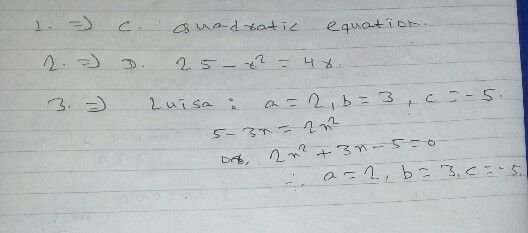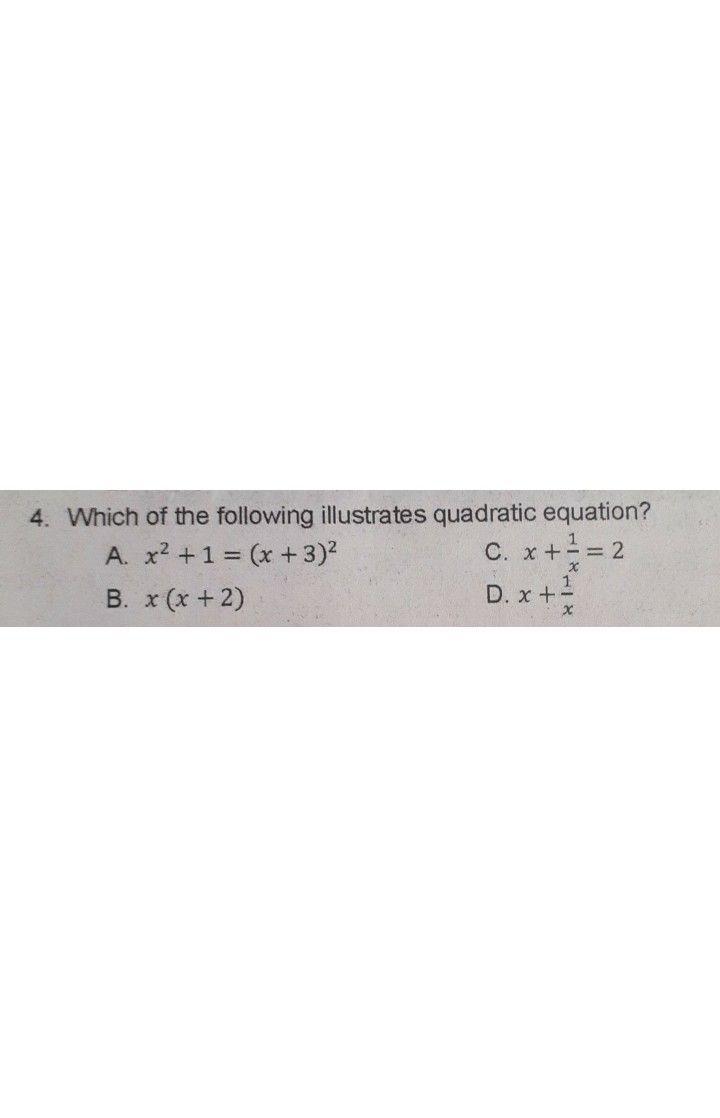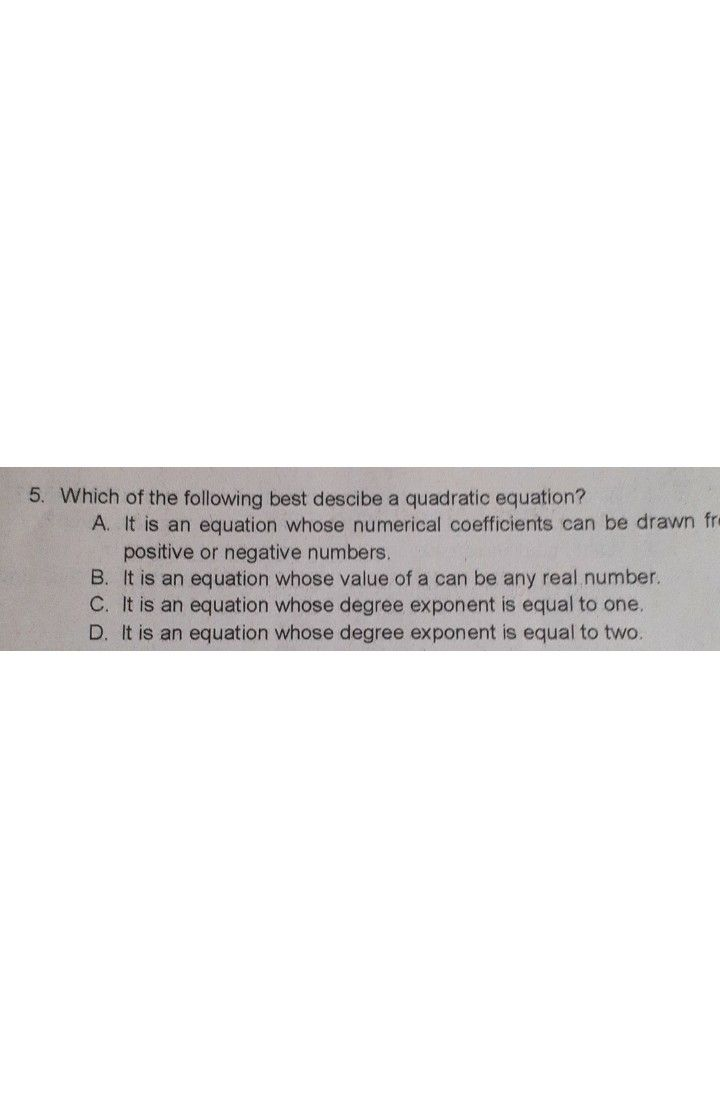Symbol
Problem$1.$ $t$ is a polynomial equation of degree two that can be written in the form $ax^{2}+bx$ $+c=0,$ where a, b, and c are real numbers and a # 0. A. Linear Equation C. Quadratic Equation B. Linear Inequality D. Quadratic Inequality 2. Which of the following illustrates quadratic equation? $\dfrac {1} {2}\left(h-6\right)=0$ A. $3m=15$ $C.\dfrac {1} {2}\left(h-$ B. $12-4x=0$ $D.$ $25-r^{2}=4r$ 3. The following are the values of a, b, and c that Edna, Luisa, Jane an Christy got when they expressed $5-3x=2x^{2}in$ standard $\left(Orm$ Edna $a=-2,b=-3,c=5$ $\sqrt{ane}$ $a=2,b=-3,c=-5$ $d1sa$ $a=2,b=3,c=-5$ $cnns5y$ $a=-2,b=-3,c=-5$
7th-9th grade
Other
Search count: 115
SolutionQanda teacher - pawanStudent